Latest Banking jobs   »   Quantitative Aptitude Quiz for IBPS 2020...

Quantitative Aptitude Quiz for IBPS 2020 Mains Exams- 6th December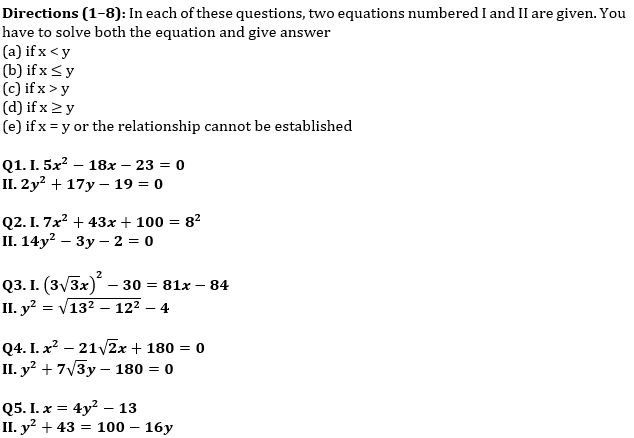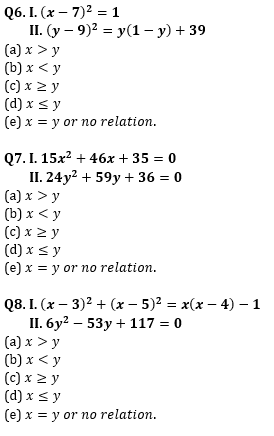Directions (9-15): In the following questions, two quantities (I) and (II) are given. You have to solve both the quantities and mark the appropriate answer.

Q9. Quantity I: Anurag invested Rs.3000 more than that of Veer, while ratio of investment period for Anurag and Veer is 5 : 6 respectively. If out of total profit of Rs.27360, Veer got Rs.12960, then find total investment done by Anurag.
Quantity II: Shivam and Deepak started a business by investing Rs.25000 and Rs.10000 respectively. At the end of the year, they decided to divide 60% of the total profit equally and rest in the investment ratio. If they had divided total profit in investment ratio, then Shivam will receive Rs.9000 more profit than that of he received actually, then find the difference between actual profit share of Shivam and that of Deepak?
(a) Quantity I < Quantity II
(b) Quantity I ≤ Quantity II
(c) Quantity I > Quantity II
(d) Quantity I ≥ Quantity II
(e) Quantity I = Quantity II or no relation.

Q10. Quantity I: Aman invested Rs.100000 at 16% p.a. The interest was compounded half yearly in the first year and in the second & third year it was compounded yearly. What will be the total interest received by man at the end of 3 years?
Quantity II: The simple interest received on a sum of Rs.2,30,000 at the rate of 23% p.a. in N years is Rs.4,23,200. Find the CI received on the same sum in (N/4) years at the rate of 11% p.a. compounded annually.
(a) Quantity I < Quantity II
(b) Quantity I ≤ Quantity II
(c) Quantity I > Quantity II
(d) Quantity I ≥ Quantity II
(e) Quantity I = Quantity II or no relation.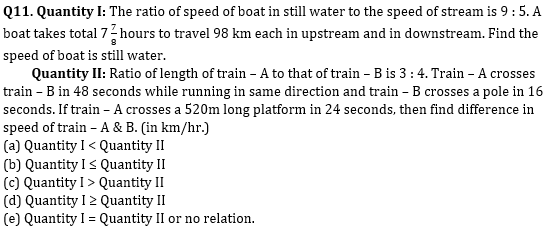Q12. Quantity I: Ratio of cost price of two articles – A & B is 5 : 6 respectively and shopkeeper marked article – A & B 40% and 25% above cost price respectively. If shopkeeper allows discount of 25% on A and 10% on B, then he get a total profit of Rs.300. Find the total cost price of article A & B.
Quantity II: Selling price of an article sold by Amit and Arun is same. Amit sold article at 20% profit and Arun sold at 25% loss. Amit calculated profit percent at cost price whereas Arun calculated loss percent at selling price. If total loss of Amit and Arun together is Rs.200. Find total cost price of Amit’s article and Arun’s article ?
(a) Quantity I < Quantity II
(b) Quantity I ≤ Quantity II
(c) Quantity I > Quantity II
(d) Quantity I ≥ Quantity II
(e) Quantity I = Quantity II or no relation.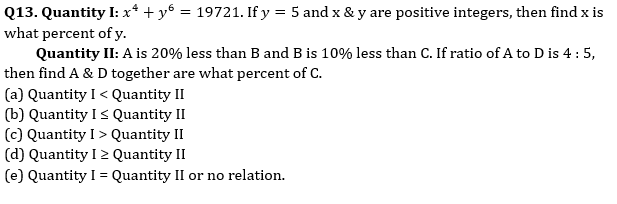Q14. In the given question, two quantities are given, one as ‘Quantity I’ and another as ‘Quantity II’. You have to determine relationship between two quantities (consider only numerical values) and choose the appropriate option.
Anurag and Veer start a business together and Veer invested 30% more amount than Anurag. Veer invested for two months more than Anurag and Anurag received Rs. 1350 as profit share out of total profit of Rs. 3690.
Quantity I – Find time for which Veer invested in the partnership.
Quantity II – If Veer invested his profit share at the rate of 7.5% p.a. for ‘t’ years at simple interest and received Rs. 1404 as interest. Find ‘t’.
(a) Quantity I ≥ Quantity II
(b) Quantity I = Quantity II or No relation
(c) Quantity I > Quantity II
(d) Quantity I < Quantity II
(e) Quantity I ≤ Quantity II

Q15. Quantity I – A bag contains four green balls, three red balls and five blue balls. If three balls taken out at random what is probability of getting at least one green ball & one blue ball.
Quantity II – There are five red toys and six green toys in a carton. If four toys are drawn at random then what is the probability of getting at least two green toys?
(a) Quantity I > Quantity II
(b) Quantity I < Quantity II
(c) Quantity I ≥ Quantity II
(d) Quantity I ≤ Quantity II
(e) Quantity I = Quantity II or no relation

Practice More Questions of Quantitative Aptitude for Competitive Exams:

Solutions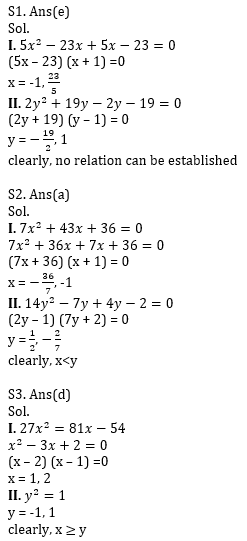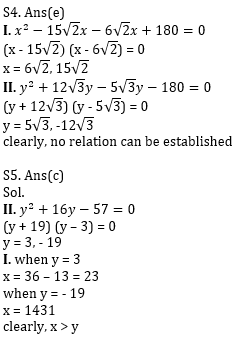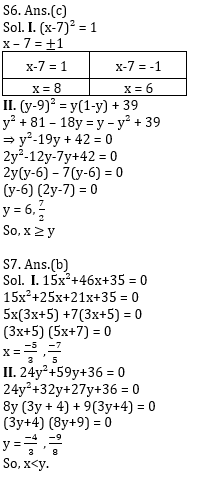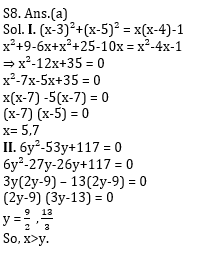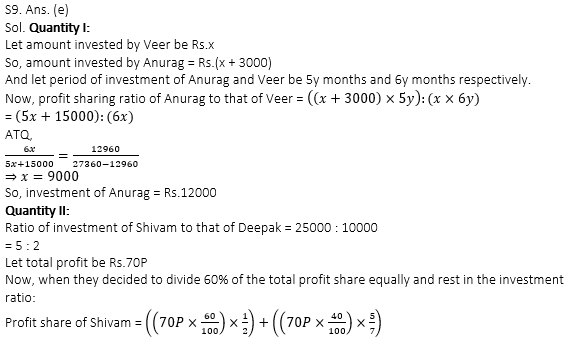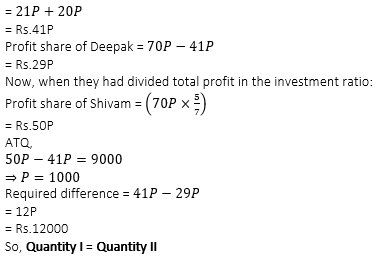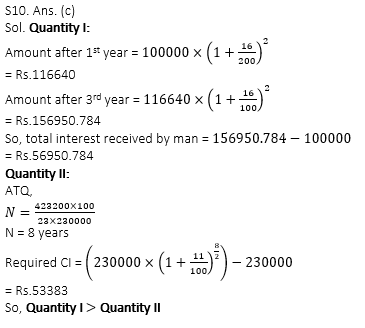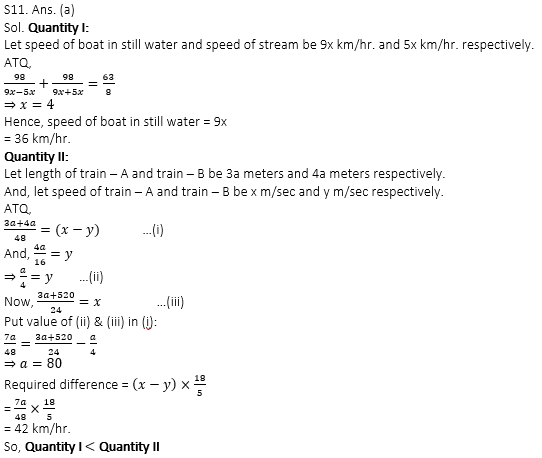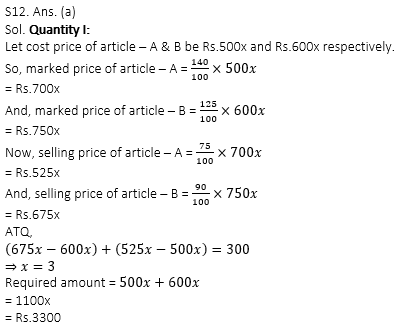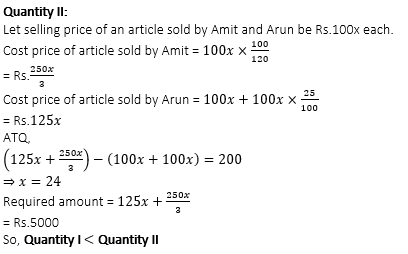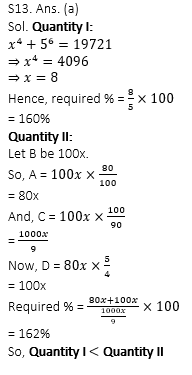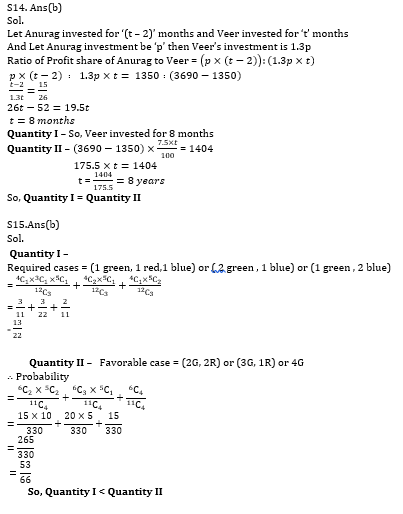Practice with Crash Course and Online Test Series for IBPS Clerk Prelims:×

Thank You, Your details have been submitted we will get back to you.Join India's largest learning destination

What You Will get ?

•Job Alerts
•Daily Quizzes
•Subject-Wise Quizzes
•Current Affairs
•Previous year question papers
•Doubt Solving session

ORJoin India's largest learning destination

What You Will get ?

•Job Alerts
•Daily Quizzes
•Subject-Wise Quizzes
•Current Affairs
•Previous year question papers
•Doubt Solving session

ORJoin India's largest learning destination

What You Will get ?

•Job Alerts
•Daily Quizzes
•Subject-Wise Quizzes
•Current Affairs
•Previous year question papers
•Doubt Solving session

Enter the email address associated with your account, and we'll email you an OTP to verify it's you.Join India's largest learning destination

What You Will get ?

•Job Alerts
•Daily Quizzes
•Subject-Wise Quizzes
•Current Affairs
•Previous year question papers
•Doubt Solving session

Enter OTP

Please enter the OTP sent to
/6

Did not recive OTP?

Resend in 60sJoin India's largest learning destination

What You Will get ?

•Job Alerts
•Daily Quizzes
•Subject-Wise Quizzes
•Current Affairs
•Previous year question papers
•Doubt Solving sessionJoin India's largest learning destination

What You Will get ?

•Job Alerts
•Daily Quizzes
•Subject-Wise Quizzes
•Current Affairs
•Previous year question papers
•Doubt Solving session

Almost there

+91Join India's largest learning destination

What You Will get ?

•Job Alerts
•Daily Quizzes
•Subject-Wise Quizzes
•Current Affairs
•Previous year question papers
•Doubt Solving session

Enter OTP

Please enter the OTP sent to Edit Number

Did not recive OTP?

Resend 60

By skipping this step you will not recieve any free content avalaible on adda247, also you will miss onto notification and job alerts

Are you sure you want to skip this step?

By skipping this step you will not recieve any free content avalaible on adda247, also you will miss onto notification and job alerts

Are you sure you want to skip this step?